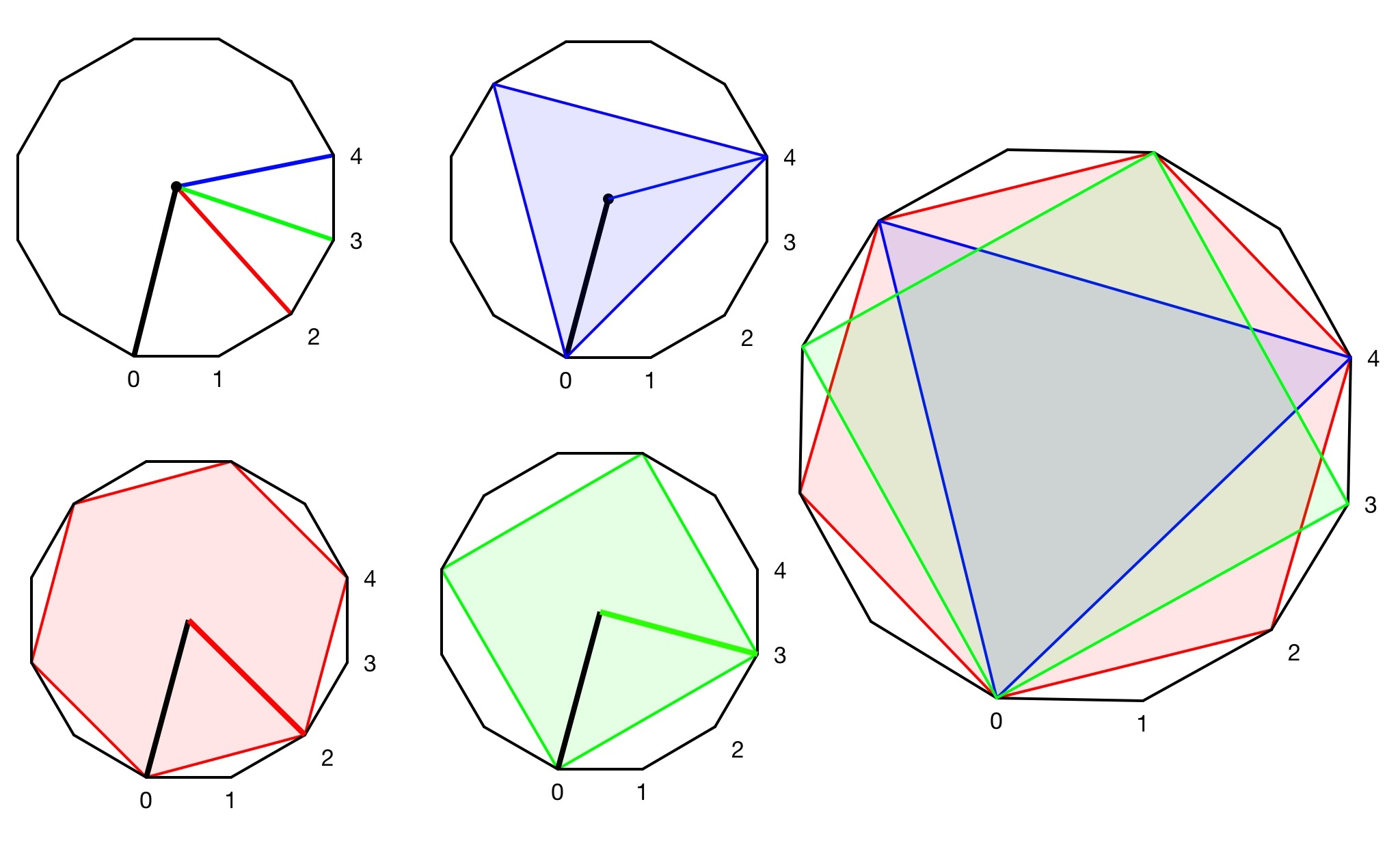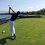# Area Created by Joining Every Kth Vertex in a Polygon$$~$$$$~$$$$~$$$$~$$$$~$$$$~$$$$~$$$$~$$$$~$$In this note, I will prove that the area formed by connecting every kth vertex of a regular polygon is $$\dfrac{a^2n(\cot{\frac{180k_i}{n}})(\csc^2{(\frac{180}{n})})(1-\cos{(\frac{k_i360}{n})})}{8k_i}$$ when $$\textbf{k divides n without remainder}$$ and n=number of sides of the original polygon and a represents the side length of the original polygon.

$~$$~$$~$$~$$~$$~$$~$$~$$~$ Can someone link me to a website so I can read up on it? I haven't been able to find one.

## Step one: determining the number of sides

$~$$~$$~$$~$$~$$~$$~$$~$$~$This step is rather simple. The number of sides of the original polygon R divided by k will yield the new polygon’s number of sides.

$~$$~$$~$$~$$~$$~$$~$$~$$~$This is because #vertices=#edges. And since we’re dividing the number of vertices by k, the number of edges will be divided by k as well.

$~$$~$$~$$~$$~$$~$$~$$~$$~$Therefore, the number of sides $=\boxed{\dfrac{n}{k_i}}$

## Step two: determining the length of each sideimage

$~$$~$$~$$~$$~$$~$$~$$~$$~$Ok, this is the hard part. For this part, I will refer to the second picture (also, NOTE 1,2,3,4 represent possible values of k). Begin by looking at the angled formed by $\theta$. It’s quite obvious that each angle is congruent. Also, $\boxed{\theta=\frac{360}{n}}$. Now, if we’re going to connect every kth vertex starting with vertex J, the angle (NOTE: point k varies in position) formed will be equal to $\boxed{\angle{JPK_i=k_i\theta}}$.

$~$$~$$~$$~$$~$$~$$~$$~$$~$Now, the one thing that remains constant no matter what vertex we chose is the length from the center of the original polygon to each vertex, AKA: the radius. The radius r is the hypotenuse of a right triangle with one leg as its apothem and the other leg being $\frac{a}{2}$. The angle at P of the right triangle is $\dfrac{\theta}{2}$. Therefore, $\boxed{r=(\frac{a}{2})\csc{(\frac{\theta}{2})}}$.

$~$$~$$~$$~$$~$$~$$~$$~$$~$Now, using Law of Co-sines, we can find M (length of segment $\overline{Jk_i}$) in terms of $k_i\theta$ and r (r is in terms of a and $\csc$ here).

$m^2=r^2+r^2-2(r)(r)\cos{(k_i\theta)}$

$m^2=2r^2(1-\cos{(k_i\theta}))$

Substituting for $r=(\frac{a}{2})\csc{(\frac{\theta}{2})}$

$m^2=2\left[(\frac{a}{2})\csc{(\frac{\theta}{2})}\right](1-\cos{(k_i\theta)})$

Substituting for $\theta=\frac{360}{n}$

$m^2=2\left[\frac{a^2\csc^2{(\frac{(\frac{360}{n})}{2})}}{4}\right](1-\cos{(k_i(\frac{360}{n})})$

$\boxed{m^2=\dfrac{a^2\csc^2{(\frac{180}{n})}(1-\cos{(\frac{360k}{n})})}{2}}$

## Finding the area

$~$$~$$~$$~$$~$$~$$~$$~$$~$This step isn't so hard, in this note, I prove that the area of any regular polygon can be represented as $\dfrac{ \left(\dfrac{n}{k_i}\right)\cot(\dfrac{180k_i}{n})m^2}{4}$. (NOTE: that I substituted a=m from the other note and the number of sides is $\dfrac{n}{k_i}$ rather than n because in this note I’m using different variables than in the other note)

Plugging in our value of $m^2$ yeilds

$area=\dfrac{\left(\dfrac{n}{k_i}\right)\cot{(\dfrac{180k_i}{n})}\left(\dfrac{a^2\csc^2{(\frac{180}{n})}(1-\cos{(\frac{360k_i}{n})})}{2}\right)}{4}$.

$area=\dfrac{ n\cot{(\dfrac{180k_i}{n})}\left(a^2\csc^2{(\frac{180}{n})}(1-\cos{(\frac{360k_i}{n})})\right)}{8k_i}$.

$\boxed{area=\dfrac{a^2n(\cot{\frac{180k_i}{n}})(\csc^2{(\frac{180}{n})})(1-\cos{(\frac{k_i360}{n})})}{8k_i}}$Note by Trevor Arashiro
6 years, 7 months ago

This discussion board is a place to discuss our Daily Challenges and the math and science related to those challenges. Explanations are more than just a solution — they should explain the steps and thinking strategies that you used to obtain the solution. Comments should further the discussion of math and science.

When posting on Brilliant:

• Use the emojis to react to an explanation, whether you're congratulating a job well done , or just really confused .
• Ask specific questions about the challenge or the steps in somebody's explanation. Well-posed questions can add a lot to the discussion, but posting "I don't understand!" doesn't help anyone.
• Try to contribute something new to the discussion, whether it is an extension, generalization or other idea related to the challenge.

MarkdownAppears as
*italics* or _italics_ italics
**bold** or __bold__ bold
- bulleted- list
• bulleted
• list
1. numbered2. list
1. numbered
2. list
Note: you must add a full line of space before and after lists for them to show up correctly
paragraph 1paragraph 2

paragraph 1

paragraph 2

[example link](https://brilliant.org)example link
> This is a quote
This is a quote
    # I indented these lines
# 4 spaces, and now they show
# up as a code block.

print "hello world"
# I indented these lines
# 4 spaces, and now they show
# up as a code block.

print "hello world"
MathAppears as
Remember to wrap math in $$ ... $$ or $ ... $ to ensure proper formatting.
2 \times 3 $2 \times 3$
2^{34} $2^{34}$
a_{i-1} $a_{i-1}$
\frac{2}{3} $\frac{2}{3}$
\sqrt{2} $\sqrt{2}$
\sum_{i=1}^3 $\sum_{i=1}^3$
\sin \theta $\sin \theta$
\boxed{123} $\boxed{123}$

Sort by:

Is this a new theorem? And can anyone build upon it such that k doesn't necessarily have to divide n?

- 6 years, 7 months ago

Probably not. You can probably use the same application of cosine rule to prove the case where k doesn't divide n; I did that when doing proving the case k = 2 for all n.

- 6 years, 7 months ago

How does your formula work? I'm plugging in $x=\cos{60}$ for a hexagon and I'm getting 25/4. But I think that it should be 1/4

- 6 years, 7 months ago

I didn't check the formula, so my formula is probably invalid :<

- 6 years, 7 months ago

If $k \nmid n$ then you cannot have a regular polygon.

- 6 years, 3 months ago

Thanks...But I am not getting it. Is there anyone who can explain me in simpler words..please?

- 6 years, 3 months ago